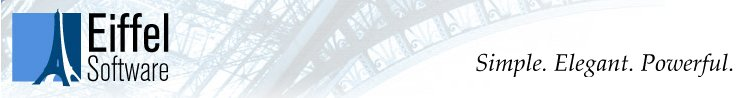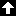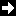This site contains older material on Eiffel. For the main Eiffel page, see http://www.eiffel.com.

### 28.10 TOWARDS A PROOF RULE

(This section is for mathematically-inclined readers only. Although you may understand the basic ideas without having had a formal exposure to the theory of programming languages, full understanding requires that you be familiar with the basics of that theory, as given for example in [M 1990], whose notations will be used here.) On first reading you may move to "DISCUSSION", 28.12, page 998.

### TO THE READER OF THIS SECTION: Sorry, the tools were not able to process the mathematical symbols properly. Treat the missing figures as an exercise; if you do need the formula, please check the published version in Object-Oriented Software Construction,

The basic mathematical property of object-oriented computation was given semi-formally in the discussion of Design by Contract:

```{INV and pre} body {INV and post}
```

where pre, post and body are the precondition, postcondition and body of a routine, and INV is the class invariant. With suitable axiomatization of the basic instructions this could serve as the basic of a fully formal axiomatic semantics for object-oriented software.

Without going that far, let us express the property more rigorously in the form of a proof rule for calls. Such a rule is fundamental for a mathematical study of O-O software since the heart of object-oriented computation is operations of the form

```t.f (..., a, ...)
```

which call a feature f, possibly with arguments such as a, on a target t representing an object. The basic proof rule may be informally stated as follows:

If we can prove that the body of f, started in a state satisfying the precondition of f, terminates in a state satisfying the postcondition, then we can deduce the same property for the above call, with actual arguments such as a substituted for the corresponding formal arguments, and every non-qualified call in the assertions (of the form some_boolean_property) replaced by the corresponding property on t (of the form t.some_boolean_ property).

For example, if we are able to prove that the actual implementation of put in class BOUNDED_QUEUE, assuming not full initially, produces a state satisfying not empty, then for any queue q and element a the rule allows us to deduce

```{not q.full} q.put (x) {not q.empty}
```

The proof rule, an adaptation to the object-oriented form of computation of Hoare's procedure proof rule may be expressed as the following inference rule:

{INV &} Body (r) {INV &}{} Call (r) {}

Here INV is the class invariant, Pre (f) is the set of precondition clauses of f and Post (f) the set of its postcondition clauses. Recall that an assertion is the conjunction of a set of clauses, of the form

```clause1; ... ; clausen
```

The large "and" signsindicate conjunction of all the clauses. The actual arguments of f have not been explicitly included in the call, but the primed expressions such as t.q' indicate substitution of the call's actual arguments for the formal arguments of f.

In the interest of conciseness, the rule is stated above in the form which does not support proofs of recursive routines. Adding such support, however, does not affect the present discussion. For details of how to handle recursion, see [M 1990].

The reason for considering the assertion clauses separately and then "anding" them is that this form prepares the rule's adaptation, described next, to separate calls in the concurrent case. Also of interest as preparation for the concurrent version is that the invariant INV must be taken into account in the proof of the routine body (above the line), with no directly visible benefit for the proof of the call (below the line). More assertions with that property will appear in the concurrent rule.

What then changes with concurrency? Waiting on a precondition clause occurs only for a precondition of the form t.cond, where t is a formal argument of the enclosing routine, and is separate. In a routine of the form

```f (..., a: T, ...) is
require
clause1; clause2; ...
do

...
end
```

any of the precondition clauses which does not involve any separate call on a separate formal argument is a correctness condition: any client must ensure that condition prior to any call, otherwise the call is in error. Any precondition clause involving a call of the form a.some_condition, where a is a separate formal argument, is a wait condition which will cause calls to block if it is not satisfied.

These observations may be expressed as a proof rule which, for separate computation, replaces the preceding sequential rule:

{INV &} Body (r) {INV &}{} Call (r) {}

where Nonsep_pre (f) is the set of clauses in f's precondition which do not involve any separate calls, and similarly for Nonsep_post (f).

This rule captures in part the essence of parallel computation. To prove a routine correct, we must still prove the same conditions (those above the line) as in the sequential rule. But the consequences on the properties of a call (below the line) are different: the client has fewer properties to ensure before the call, since, as discussed in detail earlier in this chapter, trying to ensure the separate part of the precondition would be futile anyway; but we also obtain fewer guarantees on output. The former difference may be considered good news for the client, the latter is bad news.

The separate clauses in preconditions and postconditions thus join the invariant as properties that must be included as part of the internal proof of the routine body, but are not directly usable as properties of the call.

The rule also serves to restore the symmetry between preconditions and postconditions, following a discussion that was primarily based on preconditions.Table of ContentsNext section